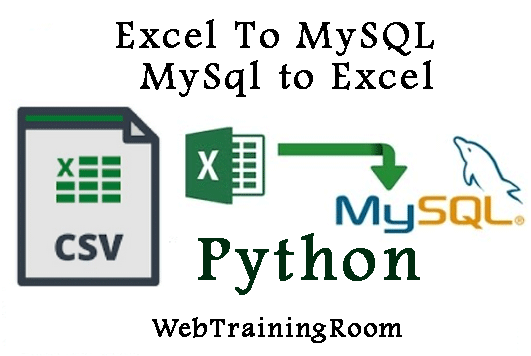# Excel to mysql using python example

In this article you will learn how to work with Excel data in python, import excel data to mysql and export mysql data to excelTo work with excel from python code we need to import following libraries

```import xlrd
import xlwt
import os
import pandas.io.sql as sql
from configparser import ConfigParser
import mysql.connector
```

You may need to install two packages for reading and writing excel, `xlrd`, `xlwt` and `pandas`

Command to install xlrd module :

```pip install xlrd
pip install xlwt
pip install pandas
```

## How to import excel data to mysql in python

In following example we read data from excel sheet and insert into mysql database

Here are the steps

1. Create a excel sheet and set some data, save the file and close
2. Keep the file in a folder under root of your project
3. Now write the following code that will insert data from excel to your database
4. Note: you need to create a custom class and then make a list of that class object with all email ids and names to make a database method. the custom class EmailInfo may look like
```class EmailInfo(object):

def __init__(self, fullname, email):
self.FullName = fullname;
self.Email = email;
```

I have created a folder called "excelFolder", Where i want to create a file with name "emailList.xlsx"

```rootPath = os.getcwd()
rootPath=rootPath+"/excelFolder/";
loc = (rootPath+"emailList.xlsx");
```

Now read the XLSX file and select the first sheet, then loop through all rows.

```wb = xlrd.open_workbook(loc)
sheet = wb.sheet_by_index(0)
for i in range(sheet.nrows):
print(sheet.cell_value(i, 0),sheet.cell_value(i, 1))
```

```def ReadFromExcel(self):
rootPath = os.getcwd()
rootPath=rootPath+"/excelFolder/";
loc = (rootPath+"emailList.xlsx");

wb = xlrd.open_workbook(loc)
sheet = wb.sheet_by_index(0)
list = [];
for i in range(sheet.nrows):
#print(sheet.cell_value(i, 0),sheet.cell_value(i, 1))
list.append(EmailInfo(sheet.cell_value(i, 0),sheet.cell_value(i, 1)));
print("Successfully retrieved all excel data");
```

The following function will insert all data into mysql database

```def BulkInsert(self,list):
mycursor = self.myConnection.cursor();
#create the table
#mycursor.execute("CREATE TABLE tbEmailList (tid INT AUTO_INCREMENT PRIMARY KEY, FullName VARCHAR(255), EmailId VARCHAR(255))");
query = "INSERT INTO tbEmailList (FullName, EmailId) VALUES ('{}', '{}')"
for obj in list:
print( obj.FullName, obj.Email);
formattedQuery=query.format(obj.FullName, obj.Email);
mycursor.execute(formattedQuery);
self.myConnection.commit()
mycursor.close()
```

### export mysql data to excel in python

You can retrieve mysql data and save that into an excel file, that can done different ways in python code.

In following example we follow the sequence below

1. Specify the file name and where to save, the full path
2. Create an instance of workbook
4. Fetch data from sql database table
5. Use `to_excel` method to save the data to that specific excel sheet

```def WriteToExcel2(self):
rootPath = os.getcwd()
rootPath=rootPath+"/excelFolder/newFile.xlsx";

workbook = xlwt.Workbook(encoding='utf-8')
worksheet.Title = "Email List";

df=sql.read_sql('SELECT firstName, LastName, RegDate FROM tbStudent',self.myConnection)
df.to_excel('ds.xls')

print("Successfully created excel file");
```

Now let's look at another example of export mysql data to excel sheet, In this example you will learn how to loop through SQL data and fill the excel worksheet and finally save in specific folder.

```def WriteToExcel(self):
rootPath = os.getcwd()
rootPath=rootPath+"/excelFolder/newFile.xlsx";

workbook = xlwt.Workbook(encoding='utf-8')
worksheet.Title = "Email List";

fileds = [u'ID',u'Name',u'Email']
for filed in range(0,len(fileds)):
worksheet.write(0,filed,fileds[filed])

workbook.save(rootPath);

print("Successfully created excel file");
```

Here is the complete code

```import xlrd
import xlwt
import os
import pandas.io.sql as sql
from configparser import ConfigParser
import mysql.connector
class ExcelExample(object):
def __init__(self):
self.Title = "Work with Excel Example";
config = ConfigParser()
database = config.get('dbinfo', 'database')
dbhost = config.get('dbinfo', 'dbhost')

self.myConnection = mysql.connector.connect(
host=config.get('dbinfo', 'dbhost'),
database=config.get('dbinfo', 'database'))
rootPath = os.getcwd()
rootPath=rootPath+"/excelFolder/";
loc = (rootPath+"emailList.xlsx");

wb = xlrd.open_workbook(loc)
sheet = wb.sheet_by_index(0)
for i in range(sheet.nrows):
print(sheet.cell_value(i, 0),sheet.cell_value(i, 1))
print("Successfully retrieved all excel data");
def WriteToExcel(self):
rootPath = os.getcwd()
rootPath=rootPath+"/excelFolder/newFile.xlsx";

workbook = xlwt.Workbook(encoding='utf-8')
worksheet.Title = "Email List";

fileds = [u'ID',u'Name',u'Email']
for filed in range(0,len(fileds)):
worksheet.write(0,filed,fileds[filed])

workbook.save(rootPath);

print("Successfully created excel file");
def WriteToExcel2(self):
rootPath = os.getcwd()
rootPath=rootPath+"/excelFolder/newFile.xlsx";

workbook = xlwt.Workbook(encoding='utf-8')